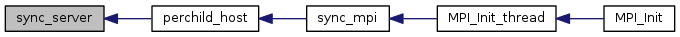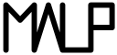Multi-ApplicationOnlineProfiling  2.1

This module is in charge of synchronizing time between MPI processes. More...

## Data Structures

struct  sync_tree_conf
This structure describes a tree node for time synchronization. More...

## Macros

#define MAX_LOOP_TRY   20
the maximum number computations tries More...

#define SYNC_TAG   1234
The tag for time sync communications. More...

#define MAX_AVG   10
the number of offset computations to perform to get an average offset More...

## Functions

void sync_mpi ()
Entry point to be used to perform time sync of every MPI Processes. More...

void compute_sync_tree (struct sync_tree_conf *conf, void(*action)(struct sync_tree_conf *next_conf, void *arg), void(*per_child_act)(struct sync_tree_conf *conf, void *arg), void(*post_action)(struct sync_tree_conf *next_conf, void *arg), void *arg)
This function does the actual work prepared by bootstrap_sync_tree(). More...

void bootstrap_sync_tree (void(*action)(struct sync_tree_conf *next_conf, void *arg), void(*per_child_act)(struct sync_tree_conf *conf, void *arg), void(*post_action)(struct sync_tree_conf *next_conf, void *arg), void *arg)
This is a bootstrapping function for calling compute_sync_tree();. More...

void perchild_host (struct sync_tree_conf *c, void *arg)
What to be done on each node's child. More...

void post_client (struct sync_tree_conf *c, void *arg)
What to be done on the client side. More...

void action_set_off (struct sync_tree_conf *c, void *poffset)
Copies offset of c to current process timer offset (poffset) More...

void action_val (struct sync_tree_conf *c, void *arg)
For debugging only. More...

void sync_server (int dest_rank)
Server function for synchronizing time. More...

void sync_client (int parent, void *poffset)
The client function for synchronizing time (computes offset between server and client) More...

static uint64_t abs_diff (uint64_t a, uint64_t b)
Computes an absolute difference. More...

static uint32_t nearest_pow (uint32_t num)
Computes the nearest. More...

## Detailed Description

This module is in charge of synchronizing time between MPI processes.

If MPI Processes are distributed onto several physical nodes, the time (see Timer ) is not consistent from a process to another.

These functions give the possibility to adjust (Process_Sync_Offset) the timers of every processes in order to have synchronized time.

## Macro Definition Documentation

 #define MAX_AVG   10

the number of offset computations to perform to get an average offset

Definition at line 53 of file Sync_Time_MPI.h.

 #define MAX_LOOP_TRY   20

the maximum number computations tries

Definition at line 43 of file Sync_Time_MPI.h.

 #define SYNC_TAG   1234

The tag for time sync communications.

Definition at line 48 of file Sync_Time_MPI.h.

## Function Documentation

 static uint64_t abs_diff ( uint64_t a, uint64_t b )
inlinestatic

Computes an absolute difference.

Parameters
 a first element b second element
Returns
the positive difference between a and b

Definition at line 192 of file Sync_Time_MPI.h.

Here is the caller graph for this function: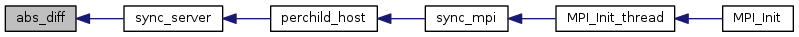void action_set_off ( struct sync_tree_conf * c, void * poffset )

Copies offset of c to current process timer offset (poffset)

Parameters
 c a node configuration poffset the current process timer offset

Definition at line 258 of file Sync_Time_MPI.c.

Here is the call graph for this function: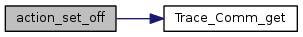Here is the caller graph for this function: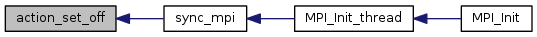void action_val ( struct sync_tree_conf * c, void * arg )

For debugging only.

Parameters
 c a node configuration arg an argument

Prints who synced with who

Definition at line 272 of file Sync_Time_MPI.c.

Here is the call graph for this function: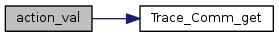void bootstrap_sync_tree ( void(*)(struct sync_tree_conf *next_conf, void *arg) action, void(*)(struct sync_tree_conf *conf, void *arg) per_child_act, void(*)(struct sync_tree_conf *next_conf, void *arg) post_action, void * arg )

This is a bootstrapping function for calling compute_sync_tree();.

Parameters
 action a function to be called on each node per_child_act a function to be called on each child of the node post_action a function to be called at the end arg an argument to give to the three above functions

The communucation is performed in a tree manner and rank 0 is the tree root.

Definition at line 93 of file Sync_Time_MPI.c.

Here is the call graph for this function: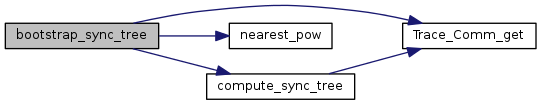Here is the caller graph for this function: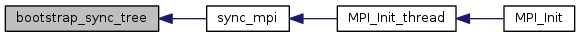void compute_sync_tree ( struct sync_tree_conf * conf, void(*)(struct sync_tree_conf *next_conf, void *arg) action, void(*)(struct sync_tree_conf *conf, void *arg) per_child_act, void(*)(struct sync_tree_conf *next_conf, void *arg) post_action, void * arg )

This function does the actual work prepared by bootstrap_sync_tree().

Parameters
 conf the sync_tree_conf corresponding to current node action a function to be called on each node per_child_act a function to be called on each child of the node post_action a function to be called at the end arg an argument to give to the three above functions

The work is :

Todo:
[DOC] describe the tree...

Definition at line 22 of file Sync_Time_MPI.c.

Here is the call graph for this function: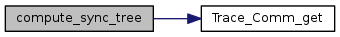Here is the caller graph for this function: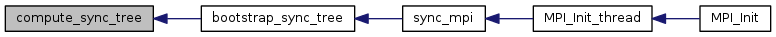static uint32_t nearest_pow ( uint32_t num )
inlinestatic

Computes the nearest.

Parameters
 num the value we want the nearest power of two
Returns
the nearest power of two that is superior to num

Definition at line 205 of file Sync_Time_MPI.h.

Here is the caller graph for this function: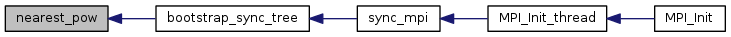void perchild_host ( struct sync_tree_conf * c, void * arg )

What to be done on each node's child.

Parameters
 c arg calls sync_server();

Definition at line 238 of file Sync_Time_MPI.c.

Here is the call graph for this function: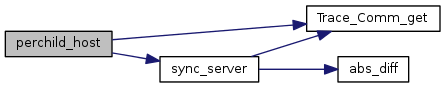Here is the caller graph for this function: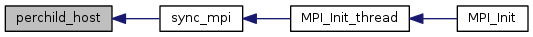void post_client ( struct sync_tree_conf * c, void * arg )

What to be done on the client side.

Parameters
 c arg calls sync_client();

Definition at line 249 of file Sync_Time_MPI.c.

Here is the call graph for this function: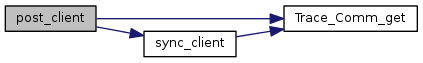Here is the caller graph for this function: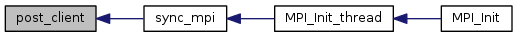void sync_client ( int parent, void * poffset )

The client function for synchronizing time (computes offset between server and client)

Parameters
 parent the parent (server) poffset the computed offset

Definition at line 204 of file Sync_Time_MPI.c.

Here is the call graph for this function: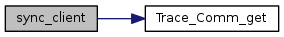Here is the caller graph for this function: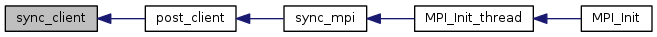void sync_mpi ( )

Entry point to be used to perform time sync of every MPI Processes.

Definition at line 279 of file Sync_Time_MPI.c.

Here is the call graph for this function: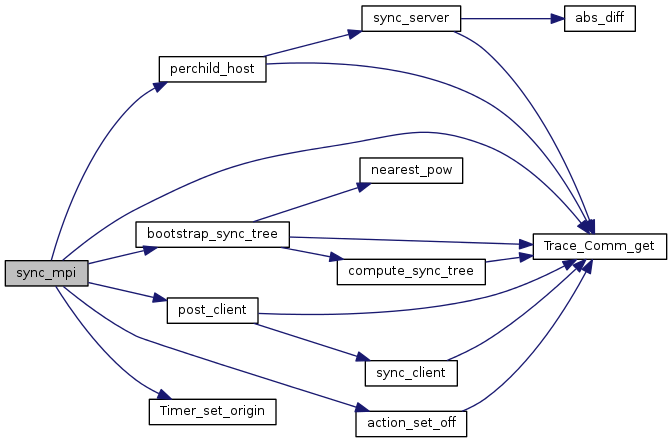Here is the caller graph for this function: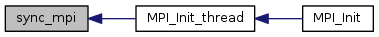void sync_server ( int dest_rank )

Server function for synchronizing time.

Parameters
 dest_rank the rank to be synchronized with self

Definition at line 132 of file Sync_Time_MPI.c.

Here is the call graph for this function: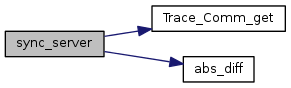Here is the caller graph for this function: